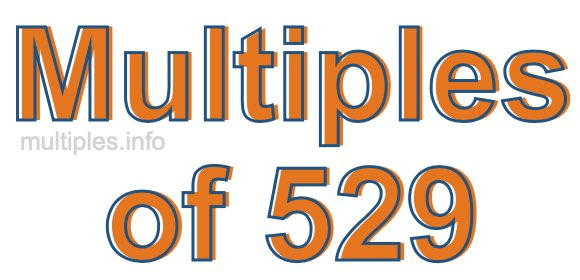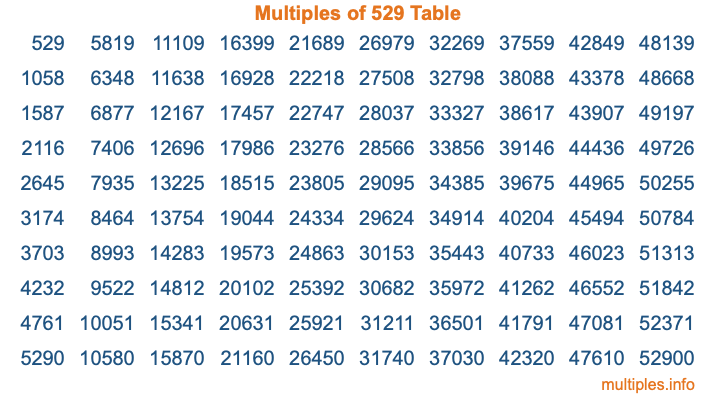Multiples of 529Welcome to the Multiples of 529 page. Here we will first teach you everything you will ever need to know about the multiples of 529, and then give you a study guide summary of everything we taught you to make sure you remember it all. Use this page to look up facts and learn information about the multiples of 529. This page will make you a multiples of five hundred twenty-nine expert!

Definition of Multiples of 529
Multiples of 529 are all the numbers that when divided by 529 equal an integer. Each of the multiples of 529 are called a multiple. A multiple of 529 is created by multiplying 529 by an integer.

Therefore, to create a list of multiples of 529, you start with 1 multiplied by 529, then 2 multiplied by 529, then 3 multiplied by 529, and so on for as long as you want. Thus, the list of the first five multiples of 529 is 529, 1058, 1587, 2116, and 2645. To see a larger list of multiples of 529, see the printable image of Multiples of 529 further down on this page. We also have a category where you can choose any nth multiple of 529.

Multiples of 529 Checker
The Multiples of 529 Checker below checks to see if any number of your choice is a multiple of 529. In other words, it checks to see if there is any number (integer) that when multiplied by 529 will equal your number. To do that, we divide your number by 529. If the the quotient is an integer, then your number is a multiple of 529.

Is  a multiple of 529?

Least Common Multiple of 529 and ...
A Least Common Multiple (LCM) is the lowest multiple that two or more numbers have in common. This is also called the smallest common multiple or lowest common multiple and is useful to know when you are adding our subtracting fractions. Enter one or more numbers below (529 is already entered) to find the LCM.

Check out our LCM Calculator if you need more details about the Least Common Multiple or if you need the LCM for different numbers for adding and subtraction fractions.

nth Multiple of 529
As we stated above, 529 is the first multiple of 529, 1058 is the second multiple of 529, 1587 is the third multiple of 529, and so on. Enter a number below to find the nth multiple of 529.

th multiple of 529

Multiples of 529 vs Factors of 529
529 is a multiple of 529 and a factor of 529, but that is where the similarities end. All postive multiples of 529 are 529 or greater than 529. All positive factors of 529 are 529 or less than 529.

Below is the beginning list of multiples of 529 and the factors of 529 so you can compare:

Multiples of 529: 529, 1058, 1587, 2116, 2645, etc.

Factors of 529: 1, 23, 529

As you can see, the multiples of 529 are all the numbers that you can divide by 529 to get a whole number. The factors of 529, on the other hand, are all the whole numbers that you can multiply by another whole number to get 529.

It's also interesting to note that if a number (x) is a factor of 529, then 529 will also be a multiple of that number (x).

Multiples of 529 vs Divisors of 529
The divisors of 529 are all the integers that 529 can be divided by evenly. Below is a list of the divisors of 529.

Divisors of 529: 1, 23, 529

The interesting thing to note here is that if you take any multiple of 529 and divide it by a divisor of 529, you will see that the quotient is an integer.

Multiples of 529 Table
Below is an image of the first 100 multiples of 529 in a table. The table is in chronological order, column by column. The first column has the first ten multiples of 529, the second column has the next ten multiples of 529, and so on.The Multiples of 529 Table is also referred to as the 529 Times Table or Times Table of 529. You are welcome to print out our table for your studies.

Negative Multiples of 529
Although not often discussed or needed in math, it is worth mentioning that you can make a list of negative multiples of 529 by multiplying 529 by -1, then by -2, then by -3, and so on, to get the following list of negative multiples of 529:

-529, -1058, -1587, -2116, -2645, etc.

Multiples of 529 Summary
Below is a summary of important Multiples of 529 facts that we have discussed on this page. To retain the knowledge on this page, we recommend that you read through the summary and explain to yourself or a study partner why they hold true.

There are an infinite number of multiples of 529.

A multiple of 529 divided by 529 will equal a whole number.

529 divided by a factor of 529 equals a divisor of 529.

The nth multiple of 529 is n times 529.

The largest factor of 529 is equal to the first positive multiple of 529.

529 is a multiple of every factor of 529.

529 is a multiple of 529.

A multiple of 529 divided by a divisor of 529 equals an integer.

529 divided by a divisor of 529 equals a factor of 529.

Any integer times 529 will equal a multiple of 529.

Multiples of a Number
Here you can get the multiples of another number, all with the same attention to detail as we did for multiples of 529 on this page.

Multiples of
Multiples of 530
Did you find our page about multiples of five hundred twenty-nine educational? Do you want more knowledge? Check out the multiples of the next number on our list!

Copyright  |   Privacy Policy  |   Disclaimer  |   Contact